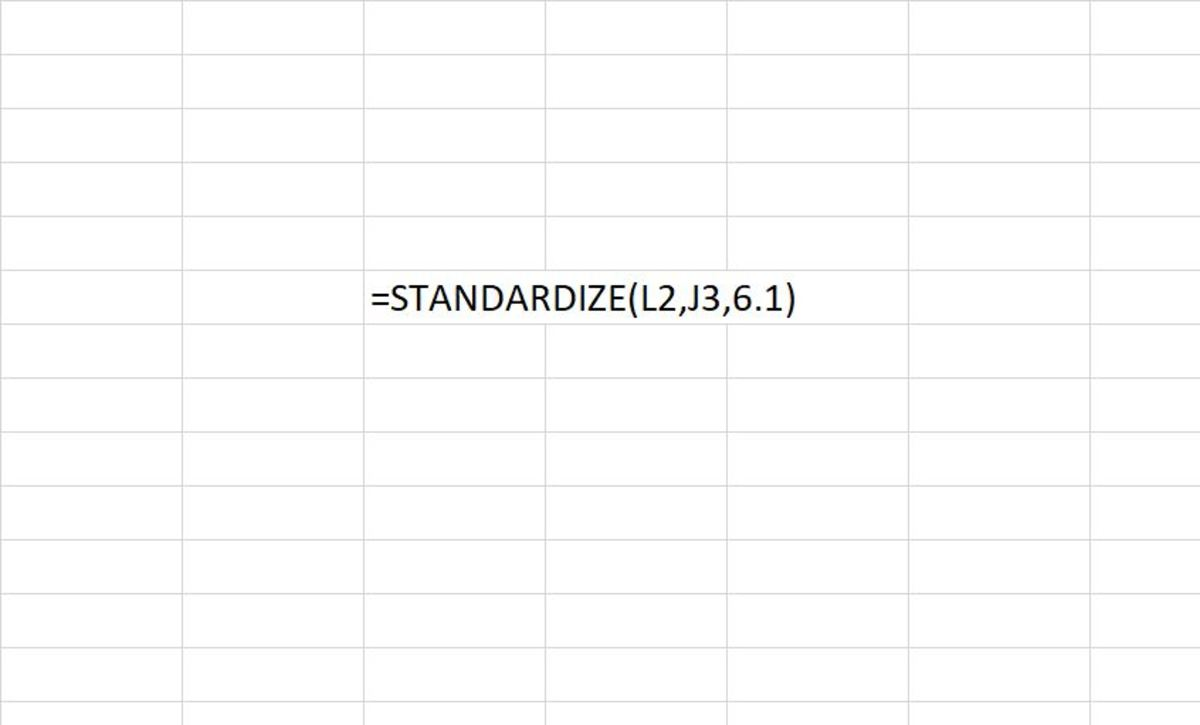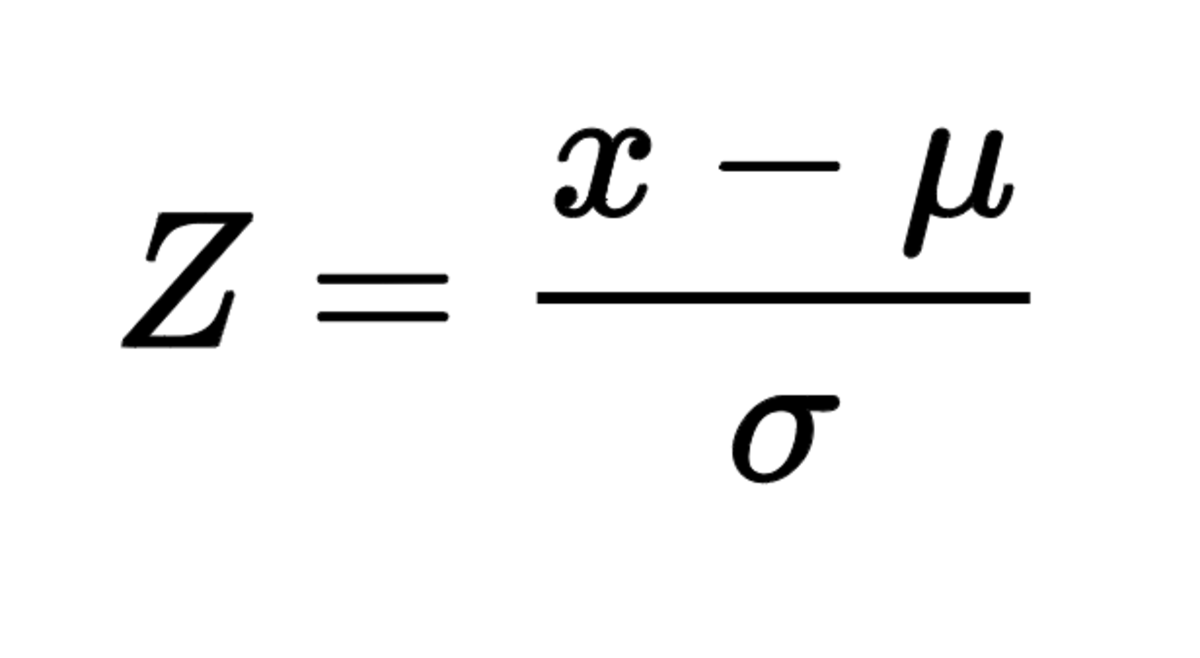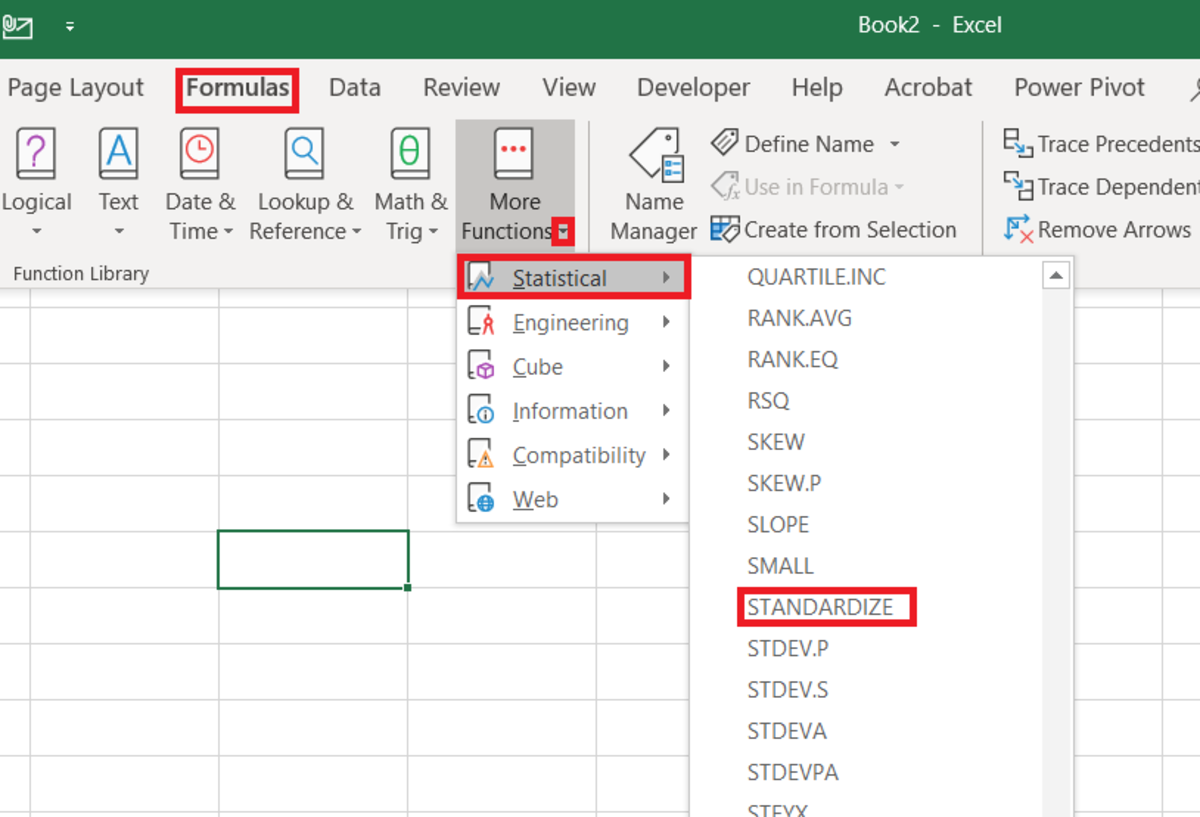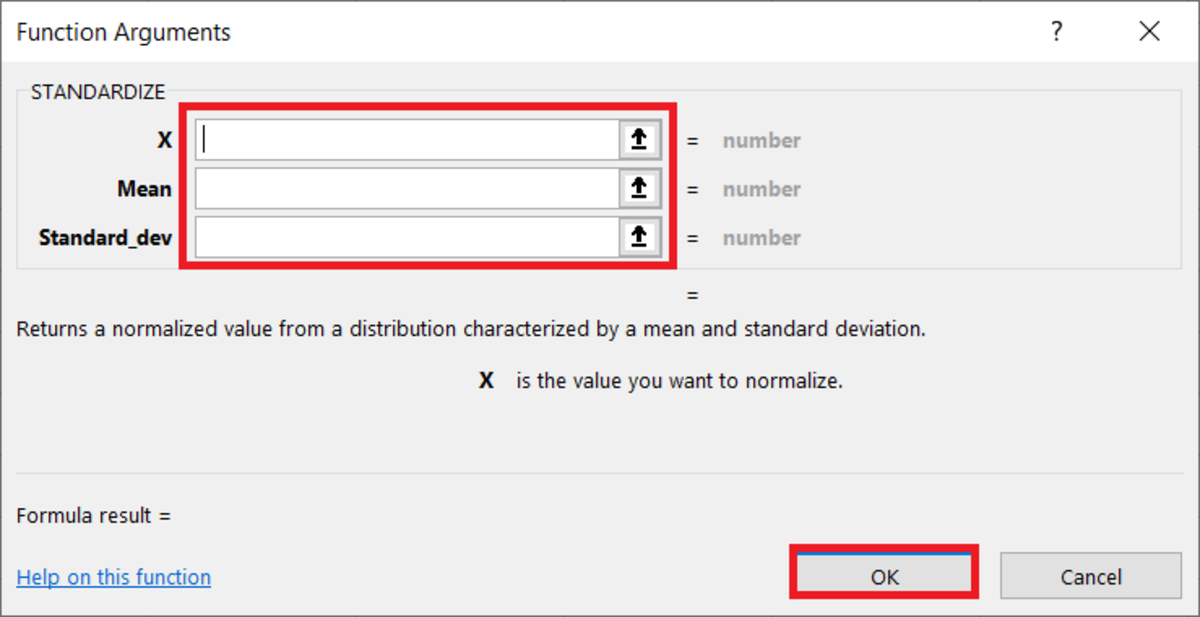# How to Use the STANDARDIZE Function in ExcelThe STANDARDIZE function is used in Excel to return a normalized value from a distribution. that is characterized by the mean and standard deviation. Pexels

Here we go through everything that you need to know to better understand the STANDARDIZE function, starting with understanding what a standardized value is and how we can use it.

## The STANDARDIZE FunctionThe STANDARDIZE function used in Microsoft Excel is a great shortcut to finding Z-scores in data sets. The function requires the variable X that represents a data point, as well as the mean and standard deviation of a data set.

## What Is the Standardized Value of a Distribution?

When one standardizes (normalizes) a value from a distribution the intention is to adjust a value of a probability distribution into alignment. When test scores are standardized, the intention would be to align the distribution of scores of students in the class to a normal distribution.

Standardized scores are useful because they allow one to find the probability of a score occurring and the ability to compare two scores from different normal distributions.

## The Purpose of the STANDARDIZE Function

The STANDARDIZE function is used to return a normalized value from a distribution as stated earlier. The normalized value that is returned is also called a Z-score. The Z-score is essentially the number of standard deviations from the population mean for a data point.

The formula in the illustration below shows exactly how the STANDARDIZE function calculates a z-score. The mean is subtracted from the number to be standardized. Then, this value is divided by the standard deviation of the data set.

## Z-Score EquationZ-scores are a popular standardized score used in the area of statistics. This method can be used when the mean and standard deviation of a population is known.

## STANDARDIZE Function Syntax

The STANDARDIZE function needs to be inputted like a formula in Excel. To manually add this formula to a cell, the following steps should be taken:

1. A cell needs to be clicked

2. "=STANDARDIZE(" needs to be typed into the cell.

3. An x value must be added followed by a comma.

4. The mean is added followed by a comma.

5. The standard deviation is added followed by a comma.

6. Finally, a close parenthesis is used at the end and the function can be entered.

The syntax is shown below followed by details on each argument that make up the STANDARDIZE function.

=STANDARDIZE(x, mean, standard_dev)

X - This is required and the value that is to be normalized.

Mean - The mean is required and better described as the arithmetic mean of the data set.

Standard_dev - Required. The standard deviation of the data set.

## Inserting the STANDARDIZE Function

The STANDARDIZE function can also be inserted into an Excel spreadsheet. This method is meant for those who are unfamiliar with the function syntax. The first steps to inserting start below.

To use this method the cell where the standardized result must display is selected. Next, the formulas tab is selected and the "More Functions" button on the Excel ribbon needs to be selected. The statistical selection from the list is then chosen followed by the STANDARDIZE selection from the drop-down menu.

## Steps to Insert the Standardize FunctionTo insert the STANDARDIZE function from the formula tab you must first navigate to: Formulas→More Functions→Statistical→STANDARDIZE

After the functions arguments window appears, values or references for each argument can be added to those fields.

The arrows on the side of the field can be used as an aid in selecting a cell reference. After the reference is selected or values are entered the OK button can be clicked.

## The Functional ArgumentsHere in the functional arguments window arguments for the STANDARDIZE function can easily be added. Instructions are given depending on which field the cursor is in so you can understand exactly what type of data needs to be added.

## STANDARDIZE Function Syntax Examples

Below are some examples with explanations of types of syntax that can be used in two examples.

=STANDARDIZE(A2,A3,A4)

Used with cell references - Here the standardized form can be found with cell references. Each reference represents data calculated from the same distribution where A2 represents x or the value being standardized, A3 represents the mean, and A4 represents the standard deviation.

=STANDARDIZE(15,14.2,1.7)

Used with numbers - Here the standardized form can be found directly with numbers added to the standardize formula. The first number 15 is the x, 14.2 is the mean, and 1.7 would be the standard deviation.

## Practical Applications for the STANDARDIZE Function

The STANDARDIZE function in Excel is useful in a variety of situations, including:

1. Data normalization: The function can be used to normalize data by converting it to a standard score, also known as a z-score. This allows for easy comparison of data points across different scales or units.
2. Statistical analysis: The function can be used to calculate the probability of a given value falling within a normal distribution. This can be useful in hypothesis testing and other statistical analysis.
3. Pattern recognition: The function can be used to identify patterns or trends in a dataset by standardizing the data and looking for deviations from the mean.
4. Quality control: The function can be used to monitor the performance of a process or system by comparing it to a set of standardized values.
5. Data visualization: The function can be used to create histograms, box plots and other graph that represent the data distribution, by standardizing the data.
6. Machine learning: The function can be useful in preprocessing data before running a model, as standardizing the data can help improve the performance of many machine learning algorithms.
7. Credit scoring: The function can be used to calculate the creditworthiness of an individual or a company by standardizing financial data, such as income and credit history.
8. Portfolio optimization: The function can be used to create a risk-adjusted return for each investment by standardizing the returns and comparing them to a benchmark.

The list above is not exhaustive, there are many other applications of the STANDARDIZE function in Excel depending on the context and the nature of the data being analyzed.

This content is accurate and true to the best of the author’s knowledge and is not meant to substitute for formal and individualized advice from a qualified professional.